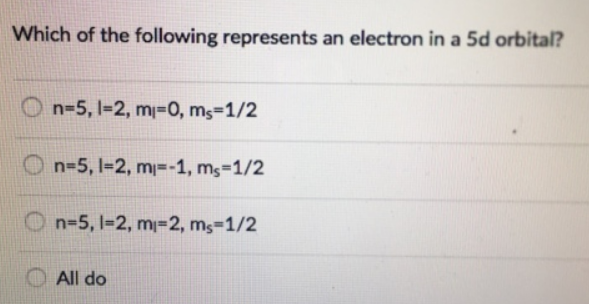# Problem: Which of the following represents an electron in a 5d orbital? (a) n=5, I=2, ml=0, ms=1/2 (b) n=5, I=2, ml=-1, ms=1/2 (c) n=5, I=2, ml=2, ms=1/2 (d) All do

###### FREE Expert Solution
94% (447 ratings)###### Problem Details

Which of the following represents an electron in a 5d orbital?

(a) n=5, I=2, ml=0, ms=1/2

(b) n=5, I=2, ml=-1, ms=1/2

(c) n=5, I=2, ml=2, ms=1/2

(d) All doFrequently Asked Questions

What scientific concept do you need to know in order to solve this problem?

Our tutors have indicated that to solve this problem you will need to apply the Quantum Numbers: Spin Quantum Number concept. You can view video lessons to learn Quantum Numbers: Spin Quantum Number. Or if you need more Quantum Numbers: Spin Quantum Number practice, you can also practice Quantum Numbers: Spin Quantum Number practice problems.

What professor is this problem relevant for?

Based on our data, we think this problem is relevant for Professor All Professors' class at HCC.